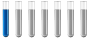## calculate mass from molar ratio

Chemistry and homework help forum.

Organic Chemistry, Analytical Chemistry, Biochemistry, Physical Chemistry, Computational Chemistry, Theoretical Chemistry, High School Chemistry, Colledge Chemistry and University Chemistry Forum.

Share your chemistry ideas, discuss chemical problems, ask for help with scientific chemistry questions, inspire others by your chemistry vision!

Please feel free to start a scientific chemistry discussion here!

Discuss chemistry homework problems with experts!

Ask for help with chemical questions and help others with your chemistry knowledge!

Moderators: expert, ChenBeier, Xen

hai
NewbiePosts: 1
Joined: Fri Oct 22, 2021 2:53 am

### calculate mass from molar ratio

Hi all

I somehow forgot the basics of m, n, and M conversions!
In x + y = xy
We have a molar ratio of x:y = 5. I know the molar masses of both compounds.
If we have 100g of xy - how to get the masses of x and y separately?
ChenBeier
Distinguished MemberPosts: 974
Joined: Wed Sep 27, 2017 7:25 am
Location: Berlin, Germany

### Re: calculate mass from molar ratio

Basic is n= m/M

If you know molar Mass M of XY then you can calculate n the moles of XY for a given mass m of 100 g and the equation reqires each component also 1 then m of X and Y also known.
If ratio X/Y = 5 then X = 5*Y but not necessary.